# One-dimensional vertex detection

When the convolution process is performed with [-1, 1], the rising part becomes a value exceeding 0 and the falling part becomes less than 0. Using this, an ascending flag and a descending flag are created. The point where the flags overlap is the apex.

``````import math
import matplotlib
import matplotlib.pyplot as plt
import numpy as np
import PIL.Image
import random
import scipy.ndimage

#One-dimensional vertex detection
x = np.array([0, 0, 1, 0, 0])
print("x", x, "Target array")
conv1 = np.convolve(x, [1, -1], mode="full")
print("conv1", conv1, "[-1, 1]Make marks for rising and falling points with the filter of")
flag1 = (conv1 > 0).astype(int)
print("flag1", flag1, "Flag of rising point")
flag2 = (conv1 <= 0).astype(int)
print("flag2", flag2, "Flag of descent point")
flag1 = flag1[:-1]
print("flag1", flag1, "Cut one end of the rising flag to match the length")
flag2 = flag2[1:]
print("flag2", flag2, "Remove one head of the descending flag to match the apex and align the length.")
flag3 = flag1 & flag2
print("flag3", flag3, "The result of ANDing the ascending flag and descending flag is the apex.")
``````

## Execution result

``````x [0 0 1 0 0]Target array
conv1 [ 0  0  1 -1  0  0] [-1, 1]Make marks for rising and falling points with the filter of
flag1 [0 0 1 0 0 0]Flag of rising point
flag2 [1 1 0 1 1 1]Flag of descent point
flag1 [0 0 1 0 0]Cut one end of the rising flag to match the length
flag2 [1 0 1 1 1]Remove one head of the descending flag to match the apex and align the length.
flag3 [0 0 1 0 0]The result of ANDing the ascending flag and descending flag is the apex.
``````

## Creating a sample

``````cycle = 4
data = np.zeros(100)
cycleWidth = len(data) / cycle
unit = math.pi / cycleWidth * 2
for i in range(cycle):
for j in range(int(cycleWidth)):
data[i * int(cycleWidth) + j] = math.cos(unit * float(j))

plt.plot(data)
plt.show()
``````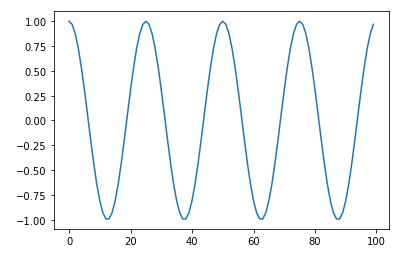## Perform vertex detection on the sample

``````#Functionize one-dimensional vertex detection
def detectPeak1D(x):
conv1 = np.convolve(x, [1, -1], mode="full")
flag1 = (conv1 > 0).astype(int)
flag2 = (conv1 <= 0).astype(int)
flag1 = flag1[:-1]
flag2 = flag2[1:]
flag3 = flag1 & flag2
return flag3

peaks = detectPeak1D(data)
plt.plot(data)
plt.plot(peaks)
plt.show()
``````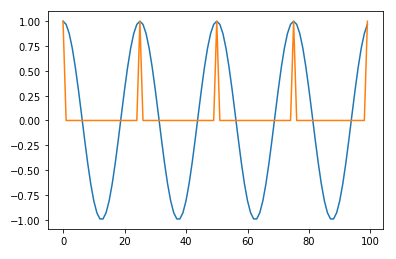## Rise is detected as a vertex for horizontal points

``````data[data > 0.7] = 0.7
peaks = detectPeak1D(data)
print("If the vertices are horizontal, the rise is detected as a vertex")
plt.plot(data)
plt.plot(peaks)
plt.show()
``````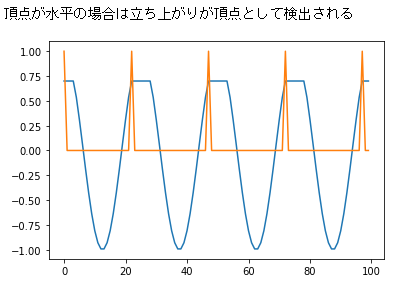# Two-dimensional vertex detection

Two-dimensional data is the result of ANDing the two "flags performed for all rows (two-dimensional array)" and "flags performed for all columns (two-dimensional array)" for one-dimensional vertex detection. To be the apex of

``````#Two-dimensional vertex detection
x = np.array([
[0, 0, 1, 0, 0],
[0, 2, 3, 2, 0],
[1, 3, 5, 3, 1],
[0, 2, 3, 2, 0],
[0, 0, 1, 0, 0],
])
print(x, "Target data")
#Perform vertex detection on every row
peaks1 = []
for ix in x:
peak = detectPeak1D(ix)
peaks1.append(peak)
peaks1 = np.array(peaks1)
print(peaks1, "Horizontal vertex detection flag")
#Perform vertex detection on all columns
peaks2 = []
for ix in x.transpose():
peak = detectPeak1D(ix)
peaks2.append(peak)
peaks2 = np.array(peaks2).transpose() #Execute detection by transpose and return to the original
print(peaks2, "Vertical vertex detection flag")
peaks3 = (peaks1 & peaks2).astype(int)
print(peaks3, "The remaining flag after ANDing the row and column detection flags becomes the two-dimensional vertex flag.")
``````

## Execution result

``````[[0 0 1 0 0]
[0 2 3 2 0]
[1 3 5 3 1]
[0 2 3 2 0]
[0 0 1 0 0]]Target data
[[0 0 1 0 0]
[0 0 1 0 0]
[0 0 1 0 0]
[0 0 1 0 0]
[0 0 1 0 0]]Horizontal vertex detection flag
[[0 0 0 0 0]
[0 0 0 0 0]
[1 1 1 1 1]
[0 0 0 0 0]
[0 0 0 0 0]]Vertical vertex detection flag
[[0 0 0 0 0]
[0 0 0 0 0]
[0 0 1 0 0]
[0 0 0 0 0]
[0 0 0 0 0]]The remaining flag after ANDing the row and column detection flags becomes the two-dimensional vertex flag.
``````
``````#Functionize two-dimensional vertex detection
def detectPeak2D(x):
peaks1 = []
for ix in x:
peak = detectPeak1D(ix)
peaks1.append(peak)
peaks1 = np.array(peaks1)
peaks2 = []
for ix in x.transpose():
peak = detectPeak1D(ix)
peaks2.append(peak)
peaks2 = np.array(peaks2).transpose()
flag = (peaks1 & peaks2).astype(int)
return flag
``````

## Creating a 2D data sample

``````#Create test data for 2D vertex detection, rotate data with 1D vertices
random.seed(1)
data2d = np.zeros((200, 200))
pattern = np.array([1, 2, 3, 4, 5, 6, 7, 6, 5, 4, 3, 2, 1])
for i in range(10):
ox, oy = random.randrange(200), random.randrange(200)
for j in range(50):
rad = (math.pi / 50) * j
for ix, v in enumerate(pattern):
pos = ix - len(pattern) / 2
x = ox + math.cos(rad) * pos
y = oy + math.sin(rad) * pos
if x < 0: continue
if x >= 200: continue
if y < 0: continue
if y >= 200: continue
data2d[int(x)][int(y)] = v

plt.figure(figsize=(10,4),dpi=200)
plt.imshow(data2d)
plt.show()
``````

## Two-dimensional sample image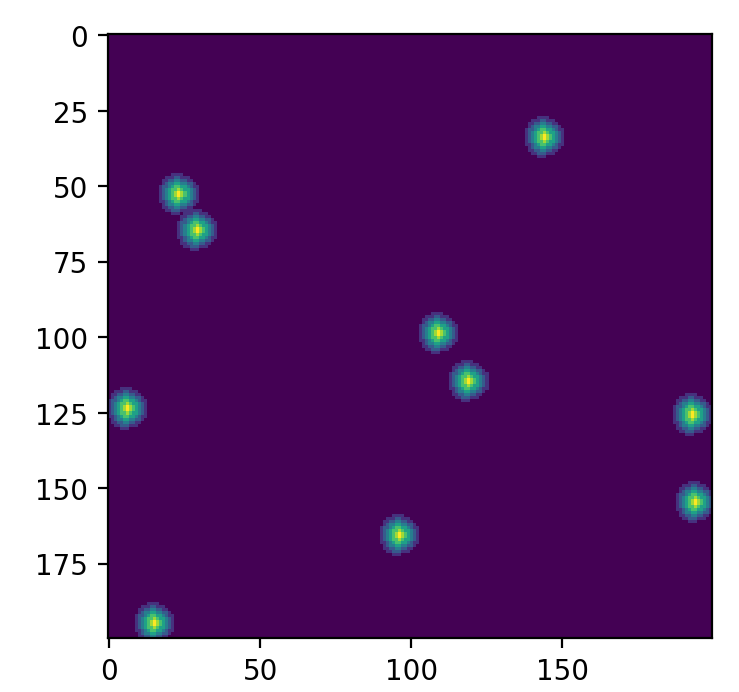## Performing 2D vertex detection

``````peaks = detectPeak2D(data2d)
print("Since all rising edges are recognized as vertices, points where the same value is continuous are recognized as vertices.")
plt.figure(figsize=(10,4),dpi=200)
plt.imshow(peaks)
plt.show()
``````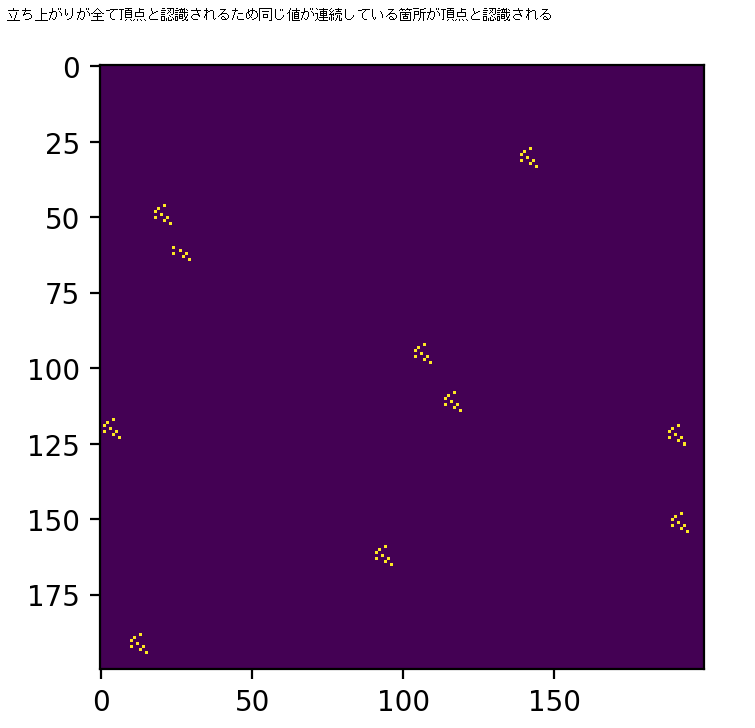## Execution of 2D vertex detection after smoothing

``````data2dGaussian = scipy.ndimage.gaussian_filter(data2d, sigma=1)
peaks = detectPeak2D(data2dGaussian)
print("Vertices can be detected normally by smoothing and eliminating parallel parts as much as possible.")
plt.figure(figsize=(10,4),dpi=200)
plt.imshow(peaks)
plt.show()
``````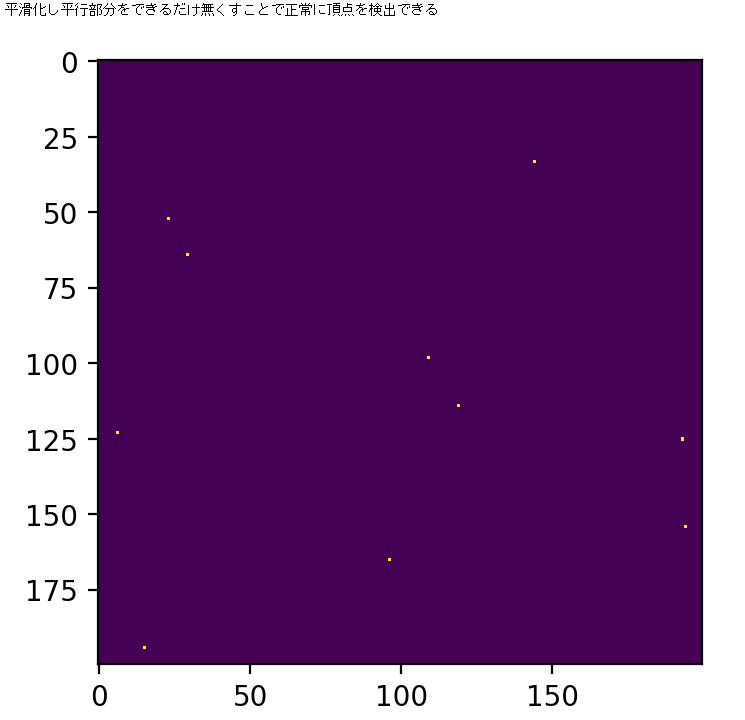# Attention etc.

There is no pre-processing or processing, and it is a process that assumes data that has a beautiful mountain shape. Basically, I think that it often does not work as expected unless it is smoothed, so use it as it is It doesn't matter if it is a moving average or a Gaussian filter, so use it after smoothing.

that's all.